## This app is for Math enthusiasts... It requires some knowledge about sine, cosine and parametric curves...

### KEY FEATURES

• Create 3D printable work of arts, jewels, study 3D surfaces and volumes....
• Generate volumes by a polygon or circle following a 3D curve.
• Generate printable u, v  surfaces.
• Save ready to 3D print STL files.
• Apply texture for  realistic rendering.
• No account needed, all processing is done locally.
After publishing (public or private), you will be able to edit your model: Animate, change color, add background, etc..

### ENTERING X, Y, Z, D, S, TW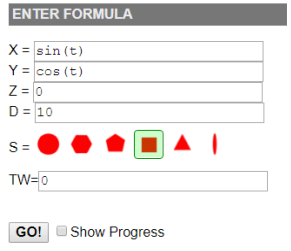The variable t defines the time from 0 to 2*pi X, Y, Z define a 3D curve as a function of t. D defines the width in mm of the generating circle/polygon as a function of time. S defines the generating shape: circle, hexagon, pentagon, square, triangle, ribbon. If S is not a circle, TW defines the twist angle of the generating shape as a function of time This formula will generate a torus: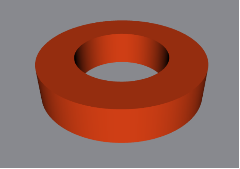Try it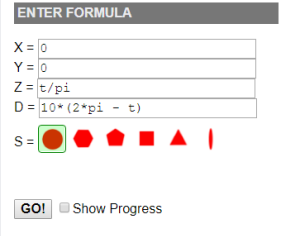D can be modified as a function of time t.This formula will generate a cone and by modifying S a variety of pyramids: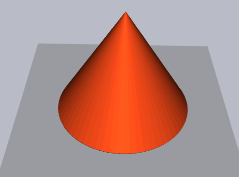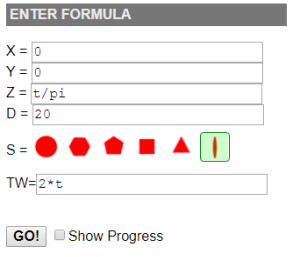If S is not a circle, TW can be used to twist the generating shape as a function of time.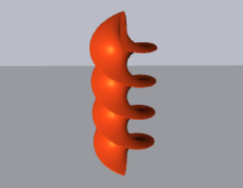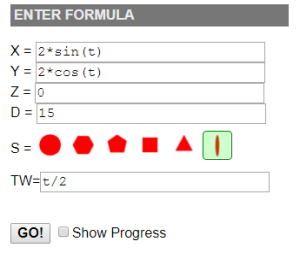The easiest way to generate a printable Mobius strip. Twist TW starts at 0 and ends at pi.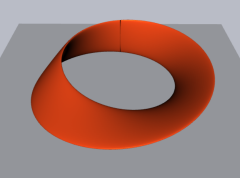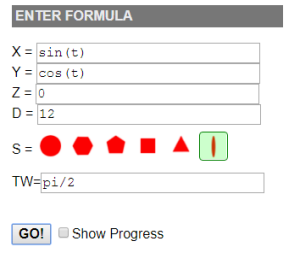Giving the ribbon a constant twist of pi/2, a ring is transformed to a washer.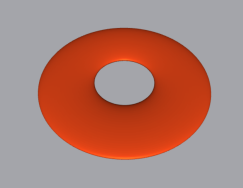### VARIABLES, OPERATORS AND FUNCTIONS

Expressions are not case sensitive.

There is a single predefined constant: pi (3.14159....)

The variable t (time) ranges initially from 0 to 2*pi

Some auxiliary variables can be used, namely:   a, b, k, r, n, w

Auxiliary variable assignment is of the form:  variable = expr;
Several assignments can be done on the same line, like
a = (t-pi)/2; b=t/(2*pi); a
Note that the last term must be the value applied to X, Y or Z...

Variables u and v are used to generate surfaces, see here.

Variables x, y, z, d can be used once they have been computed.

Following arithmetic operators can be used: + - * / < > =  +=  -=  *=  /=  <=  >=  ==  !=
and the conditional operator ? vt : vf see here.

Following functions can be used: sin(), cos(), asin(), acos(), sinh(), cosh(), abs(), exp(), log(), pow(), random(), sqrt(), tan(), atan2(), round(), floor().
pow() needs two parameters, like pow(n, 5) n to the power of 5, atan2() needs 2 parameters: y and x, random() has no parameters and generates a random number between 0 an 1.

### Examples: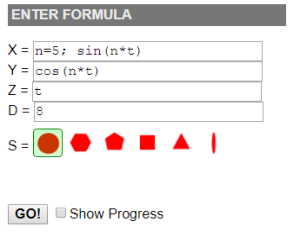Using auxiliary variable n to define turn count of a spring.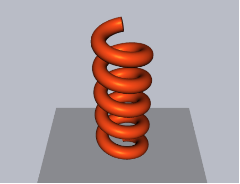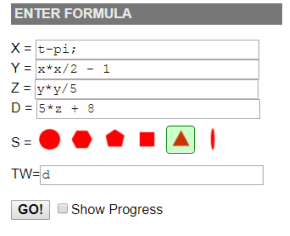Using variables x, y, z, d once they have been computed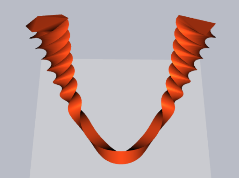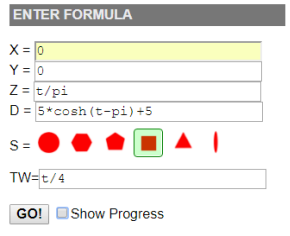Using hyperbolic function cosh.
sinh and cosh are available only on modern browsers.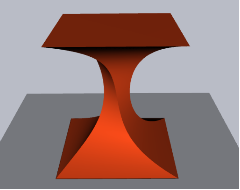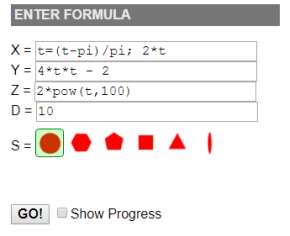Modifying t range from [0, 2*pi] to [-1, +1]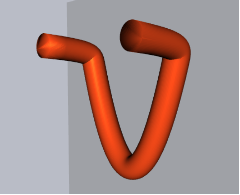### USING CONDITIONAL EXPRESSIONS

Conditional expressions are of the form: condition ? 'value if true' : 'value if false'

### Examples: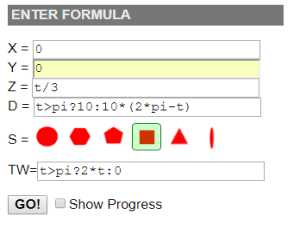Truncated pyramid with twisted handle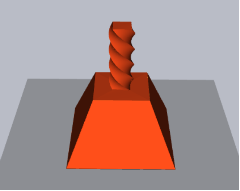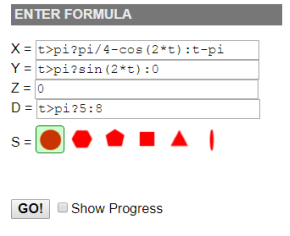Locking key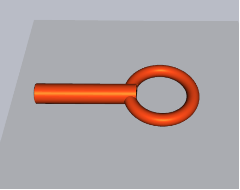### GENERATING PRINTABLE SURFACES WITH u, v VARIABLES

The app automatically generates u and v variables. u and v initially range from 0 to 2*pi. This allows defining complex u, v surfaces.
When u and v are used in the formula, a new shape control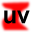is displayed. It allows displaying true 3D surfaces.
Therefore, u and v variables can be used in 2 modes:
• Pipe mode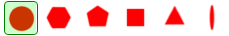:
16 values of v are used and for each v, 32 values of u are generated. The selected shape follows the X ,Y, Z 3D curve to generate a volume.
• Surface mode:
u, v are used to generate a surface. Note that in most cases, this does not generate a closed printable volume. Therefore, an additional control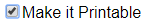is displayed. By checking it, the surface is given a 2mm thickness and becomes printable.

### Examples: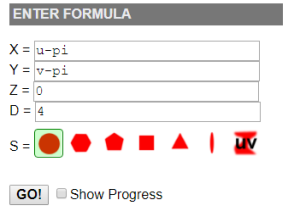A very simple u, v pipe plane. 16 pipes are generated in the Y direction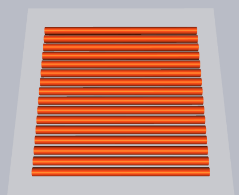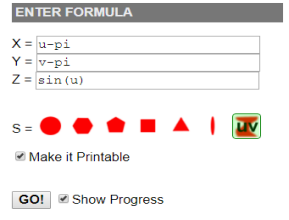A very simple printable u, v surface with 2mm thickness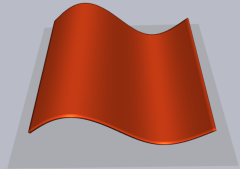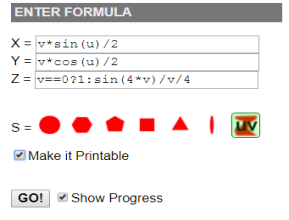Printable surface of revolution. The generating curve is Z. Note the v==0? condition to avoid 0/0 division.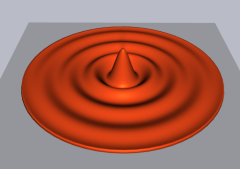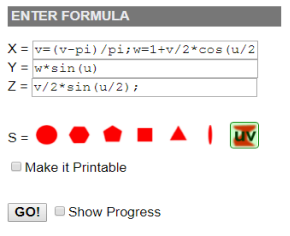A non printable Mobius strip. Note that v range is modified from [0,2*pi] to [-1,1].
There's a much easier way to define a printable Mobius strip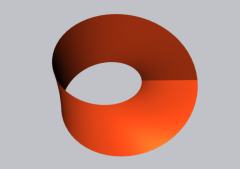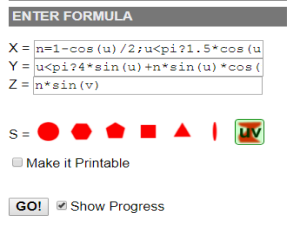A non printable Klein bottle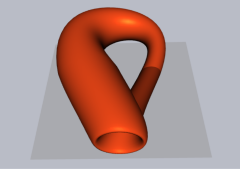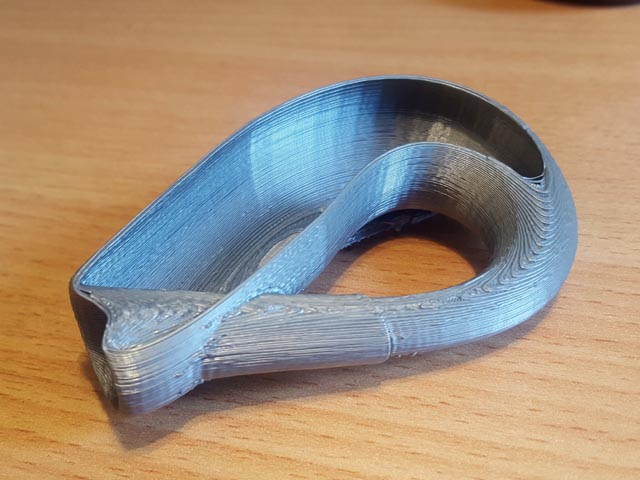Partial 3D print showing inside of Klein bottle,
using above formula with### TEXTURES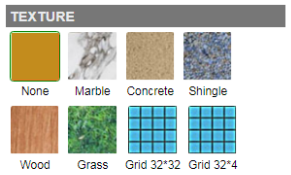Hit a texture switch for more realistic rendering. Use Grid 32*32 and Grid 32*4 to visualize volume creation. Note that STL files do not support texture. If you are the lucky owner of a full color 3D prnter or using a print service, you should first Publish your model (public, unlisted or private), then on the player page hit the Download switch. You may then save your textured model in standard OBJ format.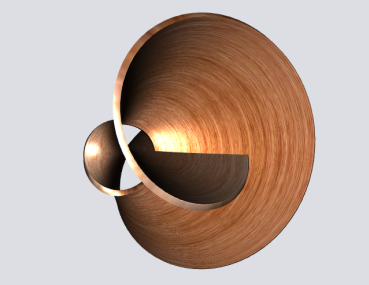A printable textured Mobius surface

 Formula expressions follow standard Javascript syntax. Therefore it is possible to create constructs like: if (condition) {statements; value;} else {statements; value} switch(expression) {case value: statements; value; break; ... case... ; default:... } The following example shows the use of "switch" to generate a tetrapod. It uses the fact that for each value of v, 32 values of u are generated. The first 4 "v" values out of 16 are used.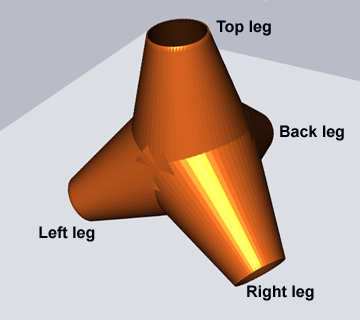X Expression:  u = u / pi / 2; // u range from 0 to 1 a = floor(v/pi*8); // a range from 0 to 15, a=0 top leg, a=1 left leg, a=2 right leg, a = 3 back leg, a>3 discard b = 1.5 * u; // scaling switch(a) {   case 0: case 3: 0; break; // top leg, back leg   case 1: -b*cos(pi/6); break; // left leg   case 2: b*cos(pi/6); break; // right leg   default: 0;} Y Expression: switch(a) {    case 0: 0; break; // top leg    case 1: case 2: -b*sin(pi/6); break; // left and right legs    case 3: b; break; // back leg    default: 0;} Z Expression: switch(a){    case 0: b; break; // top leg    case 1: case 2: case 3: -b*sin(pi/6); break; // left, right, back legs    default: 0;} D Expression: a>3 ? 0 : 8*(2.5 - b); // truncated cone, use 1.5 instead of 2.5 for full cone On the same principle, an octopod, using ribbon and twist: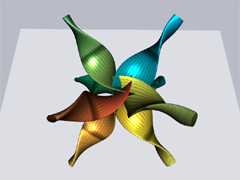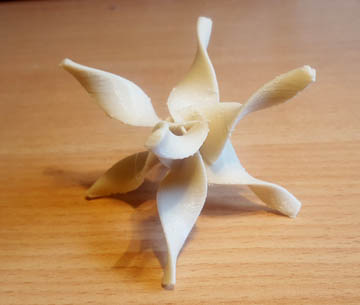Try it